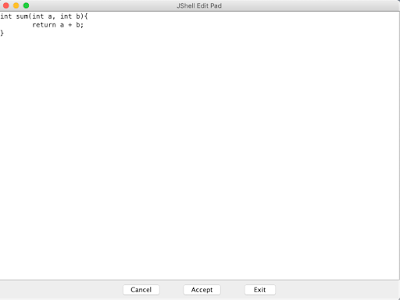# Jshell: Declare MethodsStep 1: Open terminal and execute jshell command

`\$jshell|  Welcome to JShell -- Version 10.0.2|  For an introduction type: /help introjshell>`

Step 2: Execute following statements to define sum, sub methods.

int sum(int a, int b){

return a + b;

}

int sub(int a, int b){

return a - b;

}

`jshell> int sum(int a, int b){   ...>     return a + b;   ...> }|  created method sum(int,int)jshell> jshell> int sub(int a, int b){   ...>     return a - b;   ...> }|  created method sub(int,int)jshell>`

Step 3: You can use the command /methods to list the declared methods and their signatures.

`jshell> /methods|    int sum(int,int)|    int sub(int,int)`

Step 4: Execute below statements to call sum and sub methods.

int result = sum(10, 20)

int result = sub(10, 20)

`jshell> int result = sum(10, 20)result ==> 30jshell> int result = sub(10, 20)result ==> -10`

How to update the method definition?

Step 1: Execute the command /list to see all the source that you have typed.

`jshell> /list1 : int sum(int a, int b){return a + b;       }2 : int sub(int a, int b){return a - b;       }4 : int result = sub(10, 20);`

Step 2: Numbers 1, 2 and 4 represent snippet ids. Execute the command ‘/edit 1’ to edit the definition of sum method.Update the definition of sum method like below.

int sum(int a, int b){

System.out.println("Calculating sum of " + a + " and " +  b);

return a + b;

}

Click on Accept button.

Click on Exit button.

Re execute the command /list to see whether the definition of sum is updated or not.

`jshell> /list2 : int sub(int a, int b){return a - b;       }4 : int result = sub(10, 20);5 : int sum(int a, int b){       	System.out.println("Calculating sum of " + a + " and " +  b);return a + b;       }jshell> sum(10, 20)Calculating sum of 10 and 20\$6 ==> 30`

Previous                                                    Next                                                    Home

This post first appeared on Java Tutorial : Blog To Learn Java Programming, please read the originial post: here